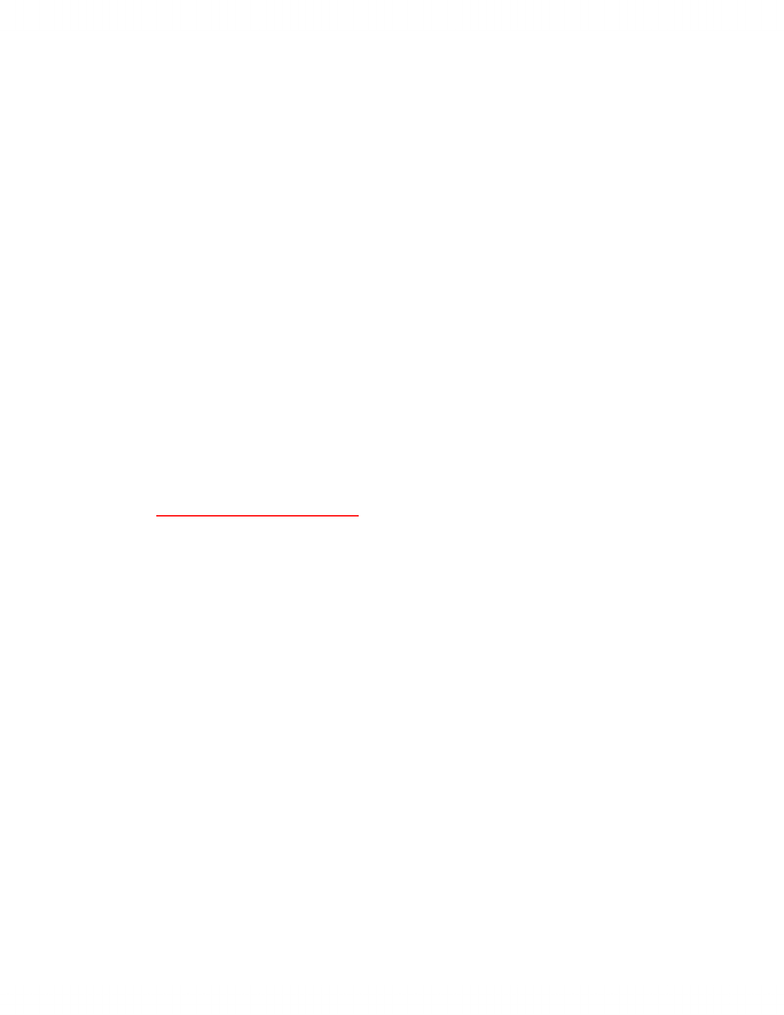Class Notes (1,100,000)
CA (650,000)
UTSG (50,000)
ECO (2,000)
Lecture

# ECO206 - assignment 1 notes

Department
Economics
Course Code
ECO206Y1
Professor
Gordon Cleveland

This preview shows pages 1-2. to view the full 6 pages of the document.TUTORIALS
Your tutorials are scheduled on the same day each week (different days for different
students). Tutorial questions are printed below.
****************************************************************
ECM A04H
TUTORIAL #1 (WEEK 2)
1. What is the opportunity cost to you of going to Economics class?
The implicit costs are my time, loss of wages from job that I could have been
doing instead. Instead of going to class I could be going out with friends or
playing. The explicit(direct) cost would be money because I paid to go to this
class.
2. What is the opportunity cost to you of getting a university education?
The implicit costs are my time and what I could have been doing instead of going
to university. I could be getting a paying job instead and earn income for the
time. The explicit costs are tuition. Must measure opportunity cost in terms of
money for both implicit and explicit costs. Measure time in dollars.
3. What is the opportunity cost to you of taking a part-time job during the school
year?
The implicit cost would be my time and the fact that I could be studying to
increased my grades for university. The explicit costs would be the money that I
make during my part time job. Value of grades dropping in dollars to compare to
the wages I make from my job.
4. Assume that Jane lives in a single-person economy and is only able to produce
juice (Y) and nuts (X). Her Production Possibilities Frontier for one month's
production can be described by the function:
Y = 400 - 30X - X20 X10
(a) What is the maximum amount of juice (in litres) that she could
produce? What is the maximum amount of nuts (in kilos) that she
could produce? The maximum number of the produce X is 10 and the
max number of produce Y is 400 (y- intercept).

Only pages 1-2 are available for preview. Some parts have been intentionally blurred.(b) Why do we restrict X to lie in the interval (0,10) when we write the
PPF as a function? We restrict X to lie in the interval (0, 10) because all
other values less than 0 are in the negative region and 10 is the
maximum number of the nut produce that are available.
(c) Is the point (5, 100) [i.e., X=5, Y=100] an efficient amount of production
for Jane? Why or why not? This is not an efficient amount of production
because when X is 5, there can be 225 units of Y. So, she is not using her
resources well.
(d) Sketch the PPF. Divide the diagram into three sets, as follows: points that
are attainable but inefficient; points that are attainable and efficient; points
that are unattainable.
(e) What is the opportunity cost of nuts at (4, 264)? What is the opportunity
cost of juice at the same point? Repeat both questions at (8,96). How are
these costs related to calculus concepts that you have learned? How are they
related to geometric properties of the graph that you have sketched?
(queston will be on exam)***
The opportunity cost of nuts = -dY/dX = - (-30 – 2x) = 30 + 2X
Opportunity cost of juice = -dX/dY = 1/(30 + 2X) (inverse)
For point (4, 264)
-dY/dX = 30 + 24 = 38L of juice
-dXdY = 1/ (30 + 2X) = 1/38 Kilos of nuts
Therefore the opportunity 38L of juice is 1/38Kilos of nuts.
For point (8, 96)
-dY/dX = 30 + 2X = 30 + 16 = 46L of juice
-dX/dY = 1/(30 + 2X) = 1/46 Kilos of nuts
The opportunity cost of 46L of juice is 1/46 kilos of nuts.
Theses costs are related to calculus concepts that I have learned because it
involves maximizing profit. They also relate to the properties of graphs because I
need to know things such as the zeros, intercepts, shapes of graphs and max/min
point. The slope of the tangent is the opportunity cost.